Updating search results...

# 25 Results

View
Selected filters:
• Algebraic ExpressionsConditional Remix & Share Permitted
CC BY-NC
Rating
5.0 stars

This unit is an EQuIP Exemplar for adult education (http://achieve.org/equip). Students will connect their prior, real-world knowledge to the concept of order in mathematics. They will go through a discovery process with content that will build a deep, conceptual understanding of the properties of operations to explain why we perform operations in a certain order when we see just the naked numbers.

Material Type:
Lesson Plan
Unit of Study
Provider:
EQuIP Exemplars
Author:
Connie Rivera
Date Added:
11/26/2014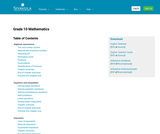Only Sharing Permitted
CC BY-ND
Rating
5.0 stars

This is a comprehensive math textbook for Grade 10. It can be downloaded, read on-line on a mobile phone, computer or iPad. Every chapter has links to on-line video lessons and explanations. Summary presentations at the end of each chapter offer an overview of the content covered, with key points highlighted for easy revision. Topics covered are: algebraic expressions, equations and inequalities, exponents, number patterns, functions, finance and growth, trigonometry, analytical geometry, statistics, probability, Euclidean geometry, measurements. This book is based upon the original Free High School Science Text series.

Subject:
Mathematics
Material Type:
Textbook
Provider:
Siyavula
Date Added:
04/12/2012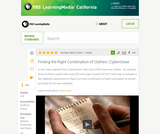Read the Fine Print
Educational Use
Rating
0.0 stars

In this video segment from Cyberchase, Harry has a fixed budget for clothing, so he must figure out what combination of jackets and pants he can buy with \$100.

Subject:
Mathematics
Algebra
Material Type:
Lecture
Provider:
PBS LearningMedia
Provider Set:
PBS Learning Media: Multimedia Resources for the Classroom and Professional Development
Author:
U.S. Department of Education
WNET
Date Added:
07/08/2008Read the Fine Print
Educational Use
Rating
0.0 stars

The CyberSquad tries to figure out how Hackerë_í__ cyberfrog moves when its various buttons are pressed, in this video from Cyberchase.

Subject:
Mathematics
Algebra
Material Type:
Lecture
Provider:
PBS LearningMedia
Provider Set:
PBS Learning Media: Multimedia Resources for the Classroom and Professional Development
Author:
U.S. Department of Education
WNET
Date Added:
07/11/2008Read the Fine Print
Educational Use
Rating
0.0 stars

In this video from Cyberchase, the CyberSquad must figure out the new input/output pattern on Hackerë_í__ larger cyberfrog.

Subject:
Mathematics
Algebra
Material Type:
Lecture
Provider:
PBS LearningMedia
Provider Set:
PBS Learning Media: Multimedia Resources for the Classroom and Professional Development
Author:
U.S. Department of Education
WNET
Date Added:
07/11/2008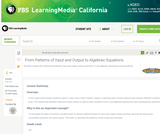Read the Fine Print
Educational Use
Rating
0.0 stars

Students explore the relationship between input and output values and learn to use algebraic expressions and equations.

Subject:
Mathematics
Algebra
Material Type:
Lesson Plan
Provider:
PBS LearningMedia
Provider Set:
PBS Learning Media Common Core Collection
Author:
U.S. Department of Education
WNET
Date Added:
10/06/2008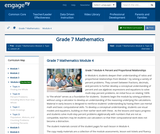Conditional Remix & Share Permitted
CC BY-NC-SA
Rating
0.0 stars

In Module 4, students deepen their understanding of ratios and proportional relationships from Module 1 by solving a variety of percent problems. They convert between fractions, decimals, and percents to further develop a conceptual understanding of percent and use algebraic expressions and equations to solve multi-step percent problems. An initial focus on relating 100% to the whole serves as a foundation for students.  Students begin the module by solving problems without using a calculator to develop an understanding of the reasoning underlying the calculations.  Material in early lessons is designed to reinforce students understanding by having them use mental math and basic computational skills. To develop a conceptual understanding, students use visual models and equations, building on their earlier work with these.  As the lessons and topics progress and students solve multi-step percent problems algebraically with numbers that are not as compatible, teachers may let students use calculators so that their computational work does not become a distraction.

Subject:
Ratios and Proportions
Material Type:
Module
Provider:
New York State Education Department
Provider Set:
EngageNY
Date Added:
01/02/2014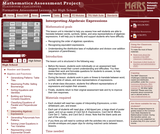Only Sharing Permitted
CC BY-NC-ND
Rating
0.0 stars

This lesson unit is intended to help teachers assess how well students are able to translate between words, symbols, tables, and area representations of algebraic expressions. It will help teachers to identify and support students who have difficulty in: recognizing the order of algebraic operations; recognizing equivalent expressions; and understanding the distributive laws of multiplication and division over addition (expansion of parentheses).

Subject:
Algebra
Material Type:
Assessment
Lesson Plan
Provider:
Shell Center for Mathematical Education
Provider Set:
Mathematics Assessment Project (MAP)
Date Added:
04/26/2013Only Sharing Permitted
CC BY-NC-ND
Rating
4.0 stars

This lesson unit is intended to help you assess how well students are able to: Perform arithmetic operations, including those involving whole-number exponents, recognizing and applying the conventional order of operations; Write and evaluate numerical expressions from diagrammatic representations and be able to identify equivalent expressions; apply the distributive and commutative properties appropriately; and use the method for finding areas of compound rectangles.

Subject:
Geometry
Material Type:
Assessment
Lesson Plan
Provider:
Shell Center for Mathematical Education
Provider Set:
Mathematics Assessment Project (MAP)
Date Added:
04/26/2013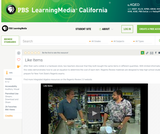Read the Fine Print
Educational Use
Rating
0.0 stars

After their carts collide in a hardware store, two teachers discover that they both bought the same items in different quantities. With limited information, this segment demonstrates how to use an equation to determine the cost of each item.

Subject:
Mathematics
Algebra
Material Type:
Lesson
Provider:
PBS LearningMedia
Provider Set:
PBS Learning Media Common Core Collection
Author:
New York Network
WNET
Date Added:
05/13/2010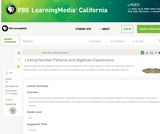Read the Fine Print
Educational Use
Rating
0.0 stars

Students practice using algebraic expressions by recording data from a video segment in which two staircases ascend at different rates. They record the patterns in two-column tables, draw line graphs and write simple algebraic relations.

Subject:
Mathematics
Algebra
Material Type:
Lesson Plan
Provider:
PBS LearningMedia
Provider Set:
PBS Learning Media Common Core Collection
Author:
U.S. Department of Education
WNET
Date Added:
07/22/2008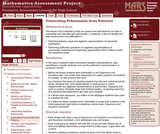Only Sharing Permitted
CC BY-NC-ND
Rating
0.0 stars

This lesson unit is intended to help you assess how well students are able to manipulate and calculate with polynomials. In particular, it aims to identify and help students who have difficulties in: switching between visual and algebraic representations of polynomial expressions; and performing arithmetic operations on algebraic representations of polynomials, factorizing and expanding appropriately when it helps to make the operations easier.

Subject:
Mathematics
Material Type:
Assessment
Lesson Plan
Provider:
Shell Center for Mathematical Education
Provider Set:
Mathematics Assessment Project (MAP)
Date Added:
04/26/2013Conditional Remix & Share Permitted
CC BY-NC
Rating
0.0 stars

Subject:
Mathematics
Material Type:
Full Course
Provider:
Pearson
Date Added:
10/06/2016Rating
0.0 stars

Expressions

Type of Unit: Concept

Prior Knowledge

Students should be able to:

Write and evaluate simple expressions that record calculations with numbers.
Use parentheses, brackets, or braces in numerical expressions and evaluate expressions with these symbols.
Interpret numerical expressions without evaluating them.

Lesson Flow

Students learn to write and evaluate numerical expressions involving the four basic arithmetic operations and whole-number exponents. In specific contexts, they create and interpret numerical expressions and evaluate them. Then students move on to algebraic expressions, in which letters stand for numbers. In specific contexts, students simplify algebraic expressions and evaluate them for given values of the variables. Students learn about and use the vocabulary of algebraic expressions. Then they identify equivalent expressions and apply properties of operations, such as the distributive property, to generate equivalent expressions. Finally, students use geometric models to explore greatest common factors and least common multiples.

Subject:
Mathematics
Algebra
Material Type:
Unit of Study
Provider:
PearsonConditional Remix & Share Permitted
CC BY-NC
Rating
0.0 stars

Students analyze how two different calculators get different values for the same numerical expression. In the process, students recognize the need for following the same conventions when evaluating expressions.Key ConceptsMathematical expressions express calculations with numbers (numerical expressions) or sometimes with letters representing numbers (algebraic expressions).When evaluating expressions that have more than one operation, there are conventions—called the order of operations—that must be followed:Complete all operations inside parentheses first.Evaluate exponents.Then complete all multiplication and division, working from left to right.Then complete all addition and subtraction, working from left to right.These conventions allow expressions with more than one operation to be evaluated in the same way by everyone. Because of these conventions, it is important to use parentheses when writing expressions to indicate which operation to do first. If there are nested parentheses, the operations in the innermost parentheses are evaluated first. Understanding the use of parentheses is especially important when interpreting the associative and the distributive properties.Goals and Learning ObjectivesEvaluate numerical expressions.Use parentheses when writing expressions.Use the order of operations conventions.

Subject:
Algebra
Material Type:
Lesson Plan
Provider:
Pearson
Date Added:
09/21/2015Conditional Remix & Share Permitted
CC BY-NC
Rating
0.0 stars

Students do a card sort in which they match expressions in words with their equivalent algebraic expressions.Key ConceptsA mathematical expression that uses letters to represent numbers is an algebraic expression.A letter used in place of a number in an expression is called a variable.An algebraic expression combines both numbers and letters using the arithmetic operations of addition (+), subtraction (–), multiplication (·), and division (÷) to express a quantity.Words can be used to describe algebraic expressions.There are conventions for writing algebraic expressions:The product of a number and a variable lists the number first with no multiplication sign. For example, the product of 5 and n is written as 5n, not n5.The product of a number and a factor in parentheses lists the number first with no multiplication sign. For example, write 5(x + 3), not (x + 3)5.For the product of 1 and a variable, either write the multiplication sign or do not write the "1." For example, the product of 1 and z is written either 1 ⋅ z or z, not 1z.Goals and Learning ObjectivesTranslate between expressions in words and expressions in symbols.

Subject:
Algebra
Material Type:
Lesson Plan
Provider:
Pearson
Date Added:
09/21/2015Conditional Remix & Share Permitted
CC BY-NC
Rating
0.0 stars

Students write an expression for the length of a train, using variables to represent the lengths of the different types of cars.Key ConceptsA numerical expression consists of a number or numbers connected by the arithmetic operations of addition, subtraction, multiplication, division, and exponentiation.An algebraic expression uses letters to represent numbers.An algebraic expression can be written to represent a problem situation. Sometimes more than one algebraic expression may represent the same problem situation. These algebraic expressions have the same value and are equivalent.The properties of operations can be used to make long algebraic expressions shorter:The commutative property of addition states that changing the order of the addends does not change the end result:a + b = b + a.The associative property of addition states that changing the grouping of the addends does not change the end result:(a + b) + c = a + (b + c).The distributive property of multiplication over addition states that multiplying a sum by a number gives the same result as multiplying each addend by the number and then adding the products together:a(b + c) = ab + ac.Goals and Learning ObjectivesWrite algebraic expressions that describe lengths of freight trains.Use properties of operations to shorten those expressions.

Subject:
Algebra
Material Type:
Lesson Plan
Provider:
Pearson
Date Added:
09/21/2015Conditional Remix & Share Permitted
CC BY-NC
Rating
0.0 stars

Students represent problem situations using expressions and then evaluate the expressions for the given values of the variables.Key ConceptsAn algebraic expression can be written to represent a problem situation.To evaluate an algebraic expression, a specific value for each variable is substituted in the expression, and then all the calculations are completed using the order of operations to get a single value.Goals and Learning ObjectivesDevelop fluency in writing expressions to represent situations and in evaluating the expressions for given values.

Subject:
Algebra
Material Type:
Lesson Plan
Provider:
Pearson
Date Added:
09/21/2015Conditional Remix & Share Permitted
CC BY-NC
Rating
0.0 stars

Four full-year digital course, built from the ground up and fully-aligned to the Common Core State Standards, for 7th grade Mathematics. Created using research-based approaches to teaching and learning, the Open Access Common Core Course for Mathematics is designed with student-centered learning in mind, including activities for students to develop valuable 21st century skills and academic mindset.

Subject:
Mathematics
Material Type:
Full Course
Provider:
Pearson
Date Added:
10/06/2016Rating
0.0 stars

Algebraic Reasoning

Type of Unit: Concept

Prior Knowledge

Students should be able to:

Add, subtract, multiply, and divide rational numbers.
Evaluate expressions for a value of a variable.
Use the distributive property to generate equivalent expressions including combining like terms.
Understand solving an equation or inequality as a process of answering a question: which values from a specified set, if any, make the equation or inequality true?
Write and solve equations of the form x+p=q and px=q for cases in which p, q, and x are non-negative rational numbers.
Understand and graph solutions to inequalities x<c or x>c.
Use equations, tables, and graphs to represent the relationship between two variables.
Relate fractions, decimals, and percents.
Solve percent problems included those involving percent of increase or percent of decrease.

Lesson Flow

This unit covers all of the Common Core State Standards for Expressions and Equations in Grade 7. Students extend what they learned in Grade 6 about evaluating expressions and using properties to write equivalent expressions. They write, evaluate, and simplify expressions that now contain both positive and negative rational numbers. They write algebraic expressions for problem situations and discuss how different equivalent expressions can be used to represent different ways of solving the same problem. They make connections between various forms of rational numbers. Students apply what they learned in Grade 6 about solving equations such as x+2=6 or 3x=12 to solving equations such as 3x+6=12 and 3(x−2)=12. Students solve these equations using formal algebraic methods. The numbers in these equations can now be rational numbers. They use estimation and mental math to estimate solutions. They learn how solving linear inequalities differs from solving linear equations and then they solve and graph linear inequalities such as −3x+4<12. Students use inequalities to solve real-world problems, solving the problem first by arithmetic and then by writing and solving an inequality. They see that the solution of the algebraic inequality may differ from the solution to the problem.

Subject:
Mathematics
Algebra
Material Type:
Unit of Study
Provider:
Pearson